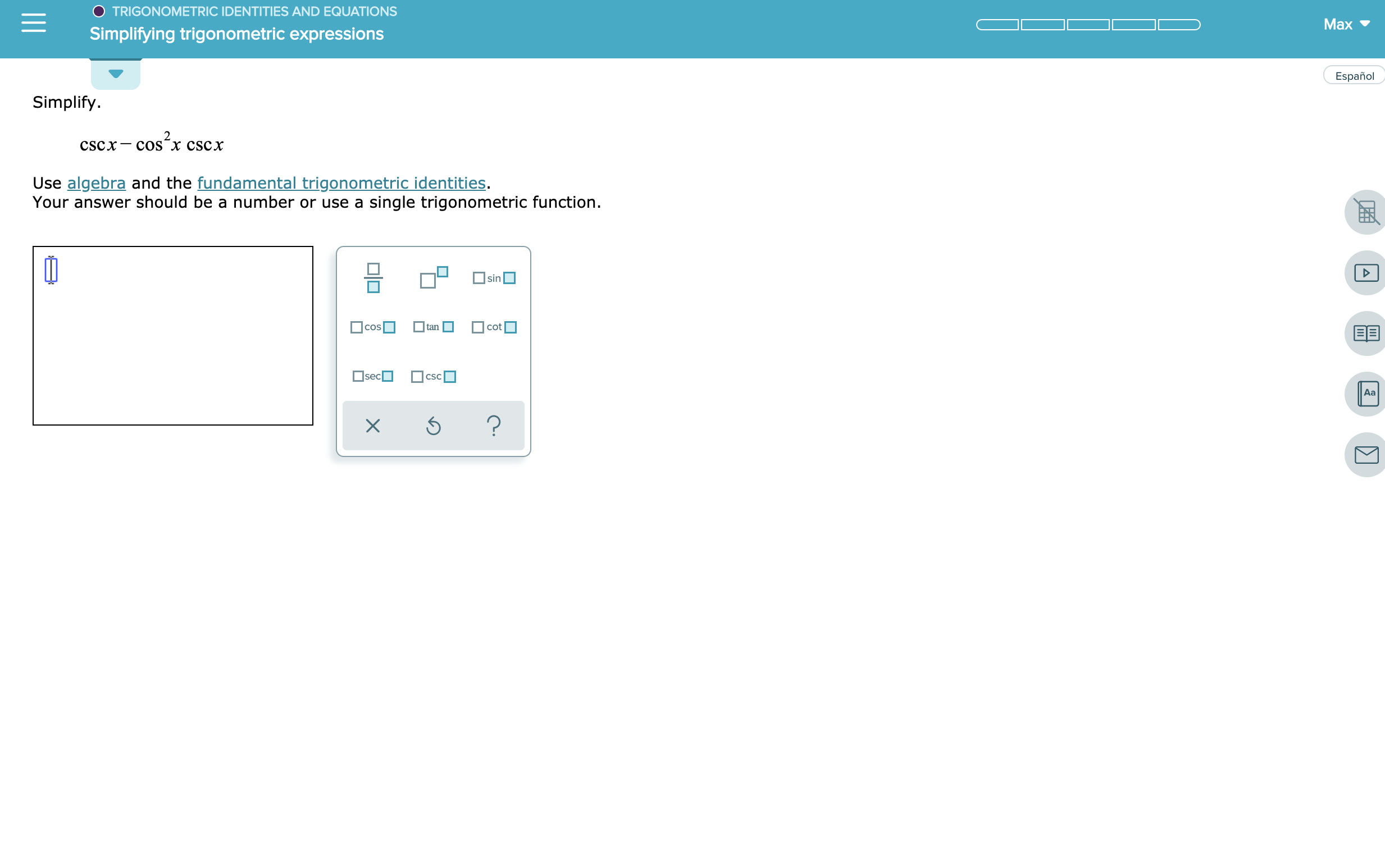# TRIGONOMETRIC IDENTITIES AND EQUATIONS Max Simplifying trigonometric expressions Español Simplify. coS"x cscх CSCX Use algebra and the fundamental trigonometric identities Your answer should be a number or use a single trigonometric function sin cot cos tan sec CSC Aa ? X

Question

See attachmenthelp_outlineImage TranscriptioncloseTRIGONOMETRIC IDENTITIES AND EQUATIONS Max Simplifying trigonometric expressions Español Simplify. coS"x cscх CSCX Use algebra and the fundamental trigonometric identities Your answer should be a number or use a single trigonometric function sin cot cos tan sec CSC Aa ? X fullscreen